# Water

Mix 68 l of water with temperature of 87 °C, 17 l warm of 42 °C and 55 l water of 50 °C.

What is the temperature of the mixed water immediately after mixing?

Result

t =  67 °C

#### Solution:Leave us a comment of example and its solution (i.e. if it is still somewhat unclear...):

Showing 0 comments:Be the first to comment!#### To solve this verbal math problem are needed these knowledge from mathematics:

Looking for help with calculating arithmetic mean? Looking for a statistical calculator? Tip: Our volume units converter will help you with converion of volume units.

## Next similar examples:

1. NoontimeIn the morning temperature was 110°F. By noontime it has gone up by 15°F. What was the noon temperature. (Give your answer in °F)
2. Temperature conversionThe normal human body temperature is 98.6 degrees Fahrenheit. What is the temperature in degrees Celcius?
3. Average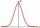Average of 7 numbers is 65. What is its sum?
4. Double percent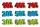What is 80% of 60% of 2800?
5. Strange x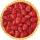For what x is true ??
6. Double 5Peter was thinking of a number. Peter doubles it and gets an answer of 8.6. What was the original number?
7. Combine / add termCombine like terms 4c+c-7c
8. AverageThe arithmetic mean of the two numbers is 71.7. One number is 5. Calculate the second number.
9. Farmer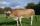The farmer farming 293 ha of arable land and the remaining 20% are meadows and pastures. How much land farmer owns?
10. EvaluateThe division of numbers 18 and 6 increase by product of the numbers 156 and 0.001
11. Find the 11Find the quotient of 229.12 and 12.32
12. Tickets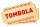On Monday, 33 tickets were sold. Every next day, twice as many as the previous day. How many tickets were sold on Friday and how many totally from Monday to Friday?
13. Percent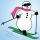From 99 children who participated in the ski course were 23 excellent skiers, 13 good and 20 average and the rest were beginners. Calculate this data in percentages.
14. 5 people5 people have \$122000 and 1 person has \$539000 How much should each person (equally) pay?
15. Family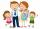Dad has two years more than mom. Mom has 5 times more than Katy. Katy has 2 times less than Jan. Jan is 10 years old. How old is everyone in the family? How old are all together?
16. Percentages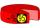Expressed as a percentage:
17. Arble bagA marble bag sold by Rachel's Marble Company contains 5 orange marbles for every 6 green marbles. If a bag has 35 orange marbles, how many green marbles does it contain?